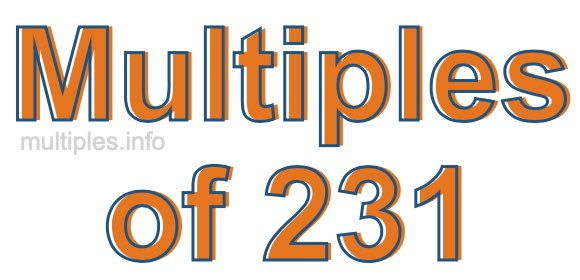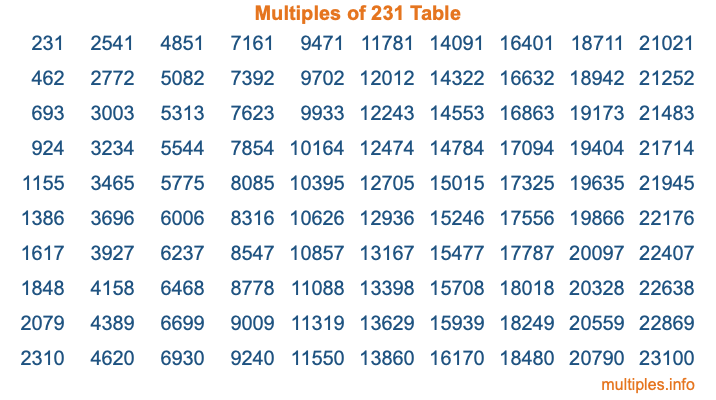Multiples of 231Welcome to the Multiples of 231 page. Here we will first teach you everything you will ever need to know about the multiples of 231, and then give you a study guide summary of everything we taught you to make sure you remember it all. Use this page to look up facts and learn information about the multiples of 231. This page will make you a multiples of two hundred thirty-one expert!

Definition of Multiples of 231
Multiples of 231 are all the numbers that when divided by 231 equal an integer. Each of the multiples of 231 are called a multiple. A multiple of 231 is created by multiplying 231 by an integer.

Therefore, to create a list of multiples of 231, you start with 1 multiplied by 231, then 2 multiplied by 231, then 3 multiplied by 231, and so on for as long as you want. Thus, the list of the first five multiples of 231 is 231, 462, 693, 924, and 1155. To see a larger list of multiples of 231, see the printable image of Multiples of 231 further down on this page. We also have a category where you can choose any nth multiple of 231.

Multiples of 231 Checker
The Multiples of 231 Checker below checks to see if any number of your choice is a multiple of 231. In other words, it checks to see if there is any number (integer) that when multiplied by 231 will equal your number. To do that, we divide your number by 231. If the the quotient is an integer, then your number is a multiple of 231.

Is  a multiple of 231?

Least Common Multiple of 231 and ...
A Least Common Multiple (LCM) is the lowest multiple that two or more numbers have in common. This is also called the smallest common multiple or lowest common multiple and is useful to know when you are adding our subtracting fractions. Enter one or more numbers below (231 is already entered) to find the LCM.

Check out our LCM Calculator if you need more details about the Least Common Multiple or if you need the LCM for different numbers for adding and subtraction fractions.

nth Multiple of 231
As we stated above, 231 is the first multiple of 231, 462 is the second multiple of 231, 693 is the third multiple of 231, and so on. Enter a number below to find the nth multiple of 231.

th multiple of 231

Multiples of 231 vs Factors of 231
231 is a multiple of 231 and a factor of 231, but that is where the similarities end. All postive multiples of 231 are 231 or greater than 231. All positive factors of 231 are 231 or less than 231.

Below is the beginning list of multiples of 231 and the factors of 231 so you can compare:

Multiples of 231: 231, 462, 693, 924, 1155, etc.

Factors of 231: 1, 3, 7, 11, 21, 33, 77, 231

As you can see, the multiples of 231 are all the numbers that you can divide by 231 to get a whole number. The factors of 231, on the other hand, are all the whole numbers that you can multiply by another whole number to get 231.

It's also interesting to note that if a number (x) is a factor of 231, then 231 will also be a multiple of that number (x).

Multiples of 231 vs Divisors of 231
The divisors of 231 are all the integers that 231 can be divided by evenly. Below is a list of the divisors of 231.

Divisors of 231: 1, 3, 7, 11, 21, 33, 77, 231

The interesting thing to note here is that if you take any multiple of 231 and divide it by a divisor of 231, you will see that the quotient is an integer.

Multiples of 231 Table
Below is an image of the first 100 multiples of 231 in a table. The table is in chronological order, column by column. The first column has the first ten multiples of 231, the second column has the next ten multiples of 231, and so on.The Multiples of 231 Table is also referred to as the 231 Times Table or Times Table of 231. You are welcome to print out our table for your studies.

Negative Multiples of 231
Although not often discussed or needed in math, it is worth mentioning that you can make a list of negative multiples of 231 by multiplying 231 by -1, then by -2, then by -3, and so on, to get the following list of negative multiples of 231:

-231, -462, -693, -924, -1155, etc.

Multiples of 231 Summary
Below is a summary of important Multiples of 231 facts that we have discussed on this page. To retain the knowledge on this page, we recommend that you read through the summary and explain to yourself or a study partner why they hold true.

There are an infinite number of multiples of 231.

A multiple of 231 divided by 231 will equal a whole number.

231 divided by a factor of 231 equals a divisor of 231.

The nth multiple of 231 is n times 231.

The largest factor of 231 is equal to the first positive multiple of 231.

231 is a multiple of every factor of 231.

231 is a multiple of 231.

A multiple of 231 divided by a divisor of 231 equals an integer.

231 divided by a divisor of 231 equals a factor of 231.

Any integer times 231 will equal a multiple of 231.

Multiples of a Number
Here you can get the multiples of another number, all with the same attention to detail as we did for multiples of 231 on this page.

Multiples of
Multiples of 232
Did you find our page about multiples of two hundred thirty-one educational? Do you want more knowledge? Check out the multiples of the next number on our list!